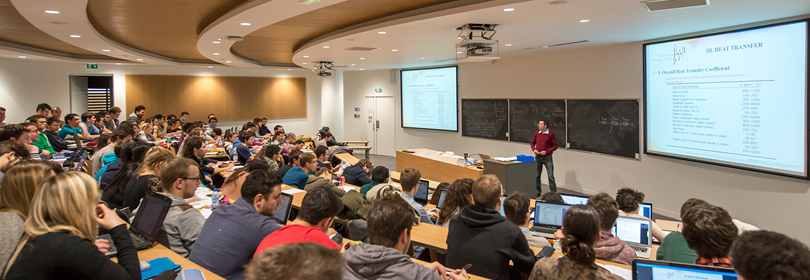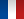Our engineering & master degrees
Ecole nationale supérieure de l'Énergie, l'Eau et l'Environnement
-
Engineering school in energy, water and environment- 3EUAFDP8

A+Augmenter la taille du texteA-Réduire la taille du texteImprimer le documentEnvoyer cette page par mail cet article Facebook Twitter Linked In
• Number of hours

• Lectures : 25.0
• Tutorials : 25.0
• Laboratory works : -
• Projects : -
• Internship : -
ECTS : 3.0
• Officials : Samuel SIEDEL

Goals

Basic notions in newtonian mechanics, electromagnetism et thermodynamics

1- Mechanics : mechanics of points and solid bodies based on Newton's 2nd law

2- Electromagnetism : Maxwell equations, physical symmetries, calculation tools and methods applied to magnetic devices in electrical engineering

3- Thermodynamique : Basic concepts of thermodynamics applied to engineering. This course is an introduction to following courses on machine thermodynamics.

Content

1-Mecanics of solid bodies
1.1- Point mechanics
Basic assumpions in mechanics
Angular momentum, energy, force, conservation laws
1.2- Solid bodies
vector derivation, velocity field of a solid body, Newto's 2nd law for a solid body
Inertia tensor, dervation of kinetic energy, linear and angular momentum
1.3- Introduction the the mechanics of systems of solid bodies
Friction and dissipation
Power, work, theorem of kinetic energy

2- Electromagnetism :
2.1- Maxwell equations and physical symmetries (4H)
2.2- Electrostatics (2H)
2.3- Tools for the study of electromagnetic devices (8H)
2.4- Diffusion of magnetic quantities (4H)

3- Thermodynamics :
Basic definitions such as system, state, equilibrium, state function, etc.
1st and 2nd principle of thermodynamics

Prerequisites

Basics of mathematics : linear algebra (matrices), differential equations, Taylor series, vector analysis

Laws of electromagnetism in vacuum

Tests

Session normale / First session
Evaluation rattrapable (ER) / ER assessment : devoir surveillé écrit de 3h (thermodynamique et électromagnétisme) / 3 hours supervised written exam (thermodynamics and electromagnetism)
Evaluation non rattrapable (EN) / EN assessment : 2 interrogations (thermodynamique et électromagnétisme) et un travail par groupe en mécanique / 2 tests (thermodynamics and electromagnetism) and a group work in mechanical eng.

Si situation 100% distancielle / If distant learning mandatory:
Evaluation rattrapable (ER) / ER assessment : examen à distance (thermodynamique et électromagnétisme) / online exam (thermodynamics and electromagnetism)
Evaluation non rattrapable (EN) / EN assessment : 2 interrogations (thermodynamique et électromagnétisme) et un travail par groupe en mécanique / 2 tests (thermodynamics and electromagnetism) and a group work in mechanical eng.

CC 2/3 + CT 1/3

Calendar

The course exists in the following branches:

• Curriculum - Master of Engineering GEE - Semester 5
see the course schedule for 2021-2022

Course language(s):